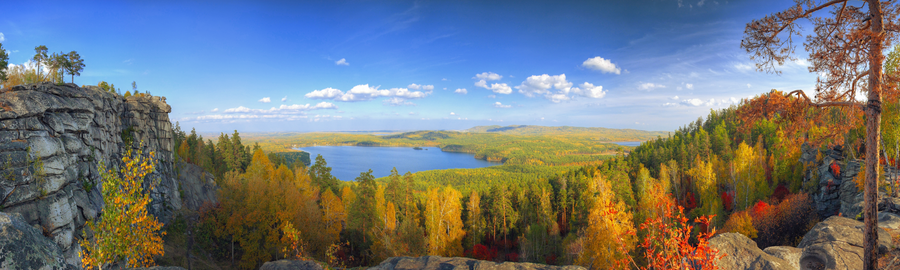# Aspect Ratio `ar`

Resizes and crops the original image to match a specified aspect ratio. This parameter will work only when either `fit=crop` or `fit=fill` is set. Values for `ar` are expressed as `W:H`.• Default: no aspect ratio set. In crop mode, the image is resized to match specified `w` and `h` dimensions exactly, discarding image data as needed. If only one dimension is specified, the other dimension is calculated automatically, preserving the original aspect ratio.

• Aspect ratio set. `ar=9:1&fit=crop`: A 900×270 image cropped using the aspect ratio of 9:1 results in an output image sized 900×100. (Note the same result can be achieved with `w=900&h=100&fit=crop`.) By default, center crop is used; see crop mode for more on directional cropping.`ar=0.85:1&fit=crop:`An image generated with the `fit=fill` and the `ar` parameter results in an image frame that is based on the specified aspect ratio and the dimensions of the original image. This acts as the maximum dimension the given image can fill. The original image is sized to fill this frame as much as possible (depending on whether the width or height of the image maxes out the frame first) while maintaining it's original aspect ratio.

In the example below, solid orange is used to fill the transparent, unused space of the image frame. For more information on fill options, please see our documentation on `fill` and `fill-color`.

`ar=9:3&fit=fill&fill=solid&fill-color=orange`:• Aspect ratio and one dimension set. `w=280&ar=2:1&fit=crop`: Using the aspect ratio of 2:1 and the specified width of 280 results in an output image sized 280×140. The `h` dimension of 140 is automatically calculated.